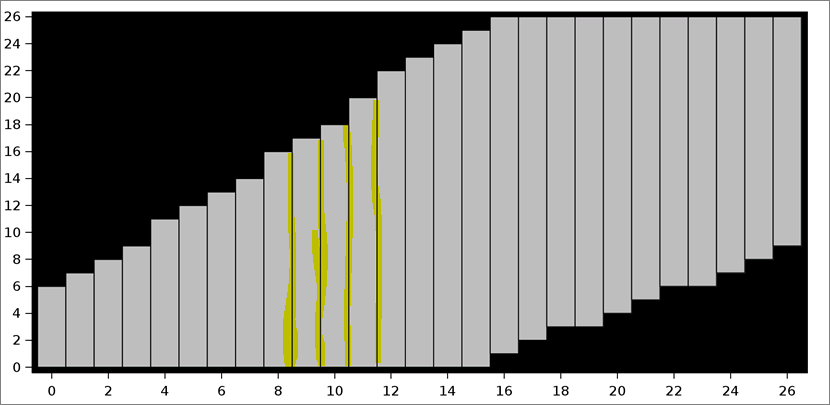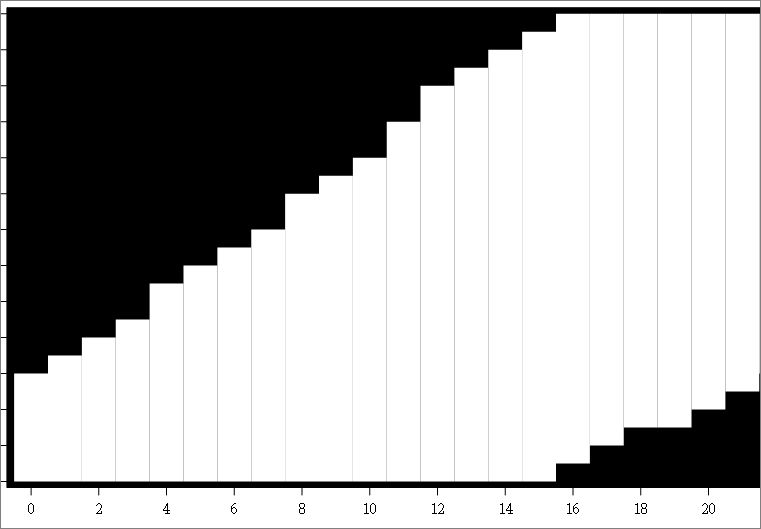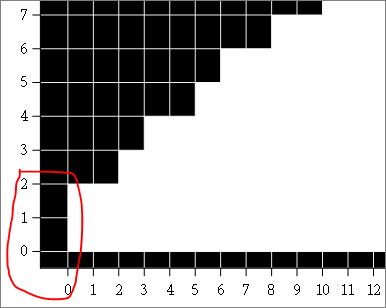## Bar Charts - remove gaps between bars

Hi,

I created this plot using sgplot-highlow with type=BAR. I want to remove the space between each bars so that i don't see the individual bars .Regards

San

1 ACCEPTED SOLUTION

Accepted SolutionsJay54
Meteorite | Level 14

## Re: Bar Charts - remove gaps between bars

RTF uses vector graphics converted into EMF+.  So, the mapping may not be perfect since you have black background (seeping through the gaps).  You may be better off using a BAND with TYPE=Step.  This will give you a continuous plot without gaps.

14 REPLIES 14

## Re: Bar Charts - remove gaps between bars

@hello28 wrote:

Hi,

I created this plot using sgplot-highlow with type=BAR. I want to remove the space between each bars so that i don't see the individual bars .Regards

San

It helps to show the code you used so we don't suggest something that conflicts with the options you have already used.

If the xaxis is descrete perhaps you want the option BARWITH=1 on the HIGHLOW statement.

## Re: Bar Charts - remove gaps between bars

proc sgplot data=plotx ;
highlow x=xbar low=ybar_low high=ybar_high /type=bar barwidth=1 dataskin= NONE fillattrs=(transparency=0.5) ;
xaxis values=(0 to 26 by 2) label='Label of X';
yaxis values=(0 to 26 by 2) label='Label of y' ;

styleattrs wallcolor=black;
run;

i need the high and low of each points connected (without showing each individual bars).

i do get this in proc gplot with plot and plot2 for high and low. however i need the wall color in black which i dont know how to get while i use gplot.Ksharp
Super User

## Re: Bar Charts - remove gaps between bars

Did you try

highlow................/ noborder

## Re: Bar Charts - remove gaps between bars

noborder is throwing a syntax error in 9.4Jay54
Meteorite | Level 14

## Re: Bar Charts - remove gaps between bars

Use NOOUTLINE.

data foo;
do i=0 to 10;
low=i+10;
high=low+5;
output;
end;
run;

proc sgplot data=foo;
highlow x=i low=low high=high / type=bar barwidth=1 nooutline;
run;

## Re: Bar Charts - remove gaps between bars

Hi Sanjay,

It Works fine in SAS Output . However when i output the image in RTF , there are some random lines.

Do you know why these are coming up?Jay54
Meteorite | Level 14

## Re: Bar Charts - remove gaps between bars

RTF uses vector graphics converted into EMF+.  So, the mapping may not be perfect since you have black background (seeping through the gaps).  You may be better off using a BAND with TYPE=Step.  This will give you a continuous plot without gaps.

## Re: Bar Charts - remove gaps between bars

Thanks Sanjay, it worked. 🙂Jay54
Meteorite | Level 14

## Re: Bar Charts - remove gaps between bars

Thanks to KSharp for follow-up post.Ksharp
Super User

## Re: Bar Charts - remove gaps between bars

Here is Sanjay suggested.

``````
data foo;
do i=0 to 10;
low=i+10;
high=low+5;
output;
end;
run;

proc sgplot data=foo;
styleattrs wallcolor=black;
band x=i lower=low upper=high / type=step   ;
run;``````

## Re: Bar Charts - remove gaps between bars

Hi,

I have a situation now.

Is it possible to plot two ranges or bands for same point on the 'x' axis. ?

From above Example: i=2; low = 12; high=14;

i=2; low = 16; high=17;Ksharp
Super User

## Re: Bar Charts - remove gaps between bars

Make two variables and two BAND statements.

i=2; low = 12; high=14;

_i=2; _low = 16; _high=17;

``band x=i lower=low upper=high / type=step   ;``
``band x=_i lower=_low upper=_high / type=step   ;``

## Re: Bar Charts - remove gaps between barsMy data is like,

x=0, y=0;

x=0, y=1;

x=0, y=2;

x=1, y=0;

x=1, y=1;

x=1, y=2;

x=2, y=0;

x=2, y=1;

x=2, y=2;

x=2, y=3;

When i use Band plot , it plots the coordinates on Xaxis and connects the next coordinates

I want to fill the grids where these points meets on x and y. For x=0 and y=0,1,2, i want to fill the three grids shown in the pictureJay54
Meteorite | Level 14

## Re: Bar Charts - remove gaps between bars

Not clear to me.  But if you want to fill the grids, you could use a HeatMap or HeatMapParm and indicate the grids that are filled and empty.  X & Y bins can be interval or discrete.  Bins can be marked at center or on edges.

You can also use HEATMAP in SGPLOT.

Also, it is preferable to start a new thread for a new issue.

Discussion stats
• 14 replies
• 2597 views
• 0 likes
• 4 in conversation